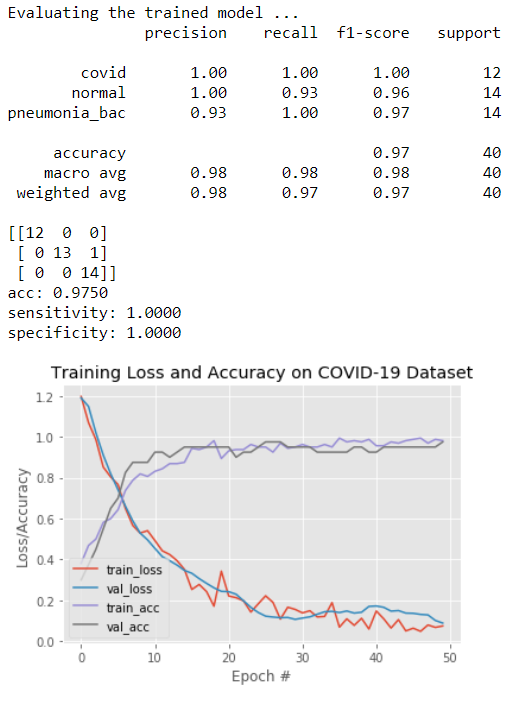# Covid-19 lung X-Ray classification and CT detection demos in 10 minutes

Original article was published on Deep Learning on Medium`# import the necessary packages from tensorflow.keras.layers import AveragePooling2D, Dropout, Flatten, Dense, Input from tensorflow.keras.models import Model from tensorflow.keras.optimizers import Adam from tensorflow.keras.utils import to_categorical from tensorflow.keras import optimizers, models, layers from tensorflow.keras.applications.inception_v3 import InceptionV3 from tensorflow.keras.applications.resnet50 import ResNet50 from tensorflow.keras.preprocessing.image import ImageDataGenerator from sklearn.preprocessing import LabelEncoder, OneHotEncoder from sklearn.model_selection import train_test_split from sklearn.metrics import classification_report, confusion_matrix from imutils import paths import matplotlib.pyplot as plt import numpy as np import cv2 import os# set learning rate, epochs and batch size INIT_LR = 1e-5 # This value is specific to what model is chosen: Inception, VGG or ResNet etc. EPOCHS = 50 BS = 8 print("Loading images...") imagePath = "./Covid_M/all/train" # change to your local path for the sample images imagePaths = list(paths.list_images(imagePath)) data = [] labels = [] # read all X-Rays in the specified path, and resize them all to 256x256 for imagePath in imagePaths: label = imagePath.split(os.path.sep)[-2] image = cv2.imread(imagePath) image = cv2.cvtColor(image, cv2.COLOR_BGR2RGB) image = cv2.resize(image, (256, 256)) data.append(image) labels.append(label) #normalise pixel values to real numbers between 0.0 - 1.0 data = np.array(data) / 255.0 labels = np.array(labels) # perform one-hot encoding for a multi-class labeling label_encoder = LabelEncoder() integer_encoded = label_encoder.fit_transform(labels) labels = to_categorical(integer_encoded) print("... ... ", len(data), "images loaded in multiple classes:") print(label_encoder.classes_)Loading images... ... ... 200 images loaded in 3x classes: ['covid' 'normal' 'pneumonia_bac']`

# 3. Add in basic data augment, re-compose the model, then train it

`# split the data between train and validation. (trainX, testX, trainY, testY) = train_test_split(data, labels, test_size=0.20, stratify=labels, random_state=42) # add on a simple Augmentation. Note: too many Augumentation doesn't actually help in this case - I found during the test. trainAug = ImageDataGenerator(rotation_range=15, fill_mode="nearest") #Use the InveptionV3 model with Transfer Learning of pre-trained "ImageNet"'s weights. #note: If you choose VGG16 or ResNet you may need to reset the initial learning rate at the top. baseModel = InceptionV3(weights="imagenet", include_top=False, input_tensor=Input(shape=(256, 256, 3))) #baseModel = VGG16(weights="imagenet", include_top=False, input_tensor=Input(shape=(256, 256, 3))) #baseModel = ResNet50(weights="imagenet", include_top=False, input_tensor=Input(shape=(256, 256, 3))) #Add on a couple of custom CNN layers on top of the Inception V3 model. headModel = baseModel.output headModel = AveragePooling2D(pool_size=(4, 4))(headModel) headModel = Flatten(name="flatten")(headModel) headModel = Dense(64, activation="relu")(headModel) headModel = Dropout(0.5)(headModel) headModel = Dense(3, activation="softmax")(headModel) # Compose the final model model = Model(inputs=baseModel.input, outputs=headModel) # Unfreeze pre-trained Inception "ImageNet" weights for re-training since I got a Navidia T4 GPU to play with anyway #for layer in baseModel.layers: # layer.trainable = False print("Compiling model...") opt = Adam(lr=INIT_LR, decay=INIT_LR / EPOCHS) model.compile(loss="categorical_crossentropy", optimizer=opt, metrics=["accuracy"]) # train the full model, since we unfroze the pre-trained weights above print("Training the full stack model...") H = model.fit_generator( trainAug.flow(trainX, trainY, batch_size=BS), steps_per_epoch=len(trainX) // BS, validation_data=(testX, testY), validation_steps=len(testX) // BS, epochs=EPOCHS)... ... Compiling model... Training the full stack model... ... ... Use tf.cast instead. Epoch 1/50 40/40 [==============================] - 1s 33ms/sample - loss: 1.1898 - acc: 0.3000 20/20 [==============================] - 16s 800ms/step - loss: 1.1971 - acc: 0.3812 - val_loss: 1.1898 - val_acc: 0.3000 Epoch 2/50 40/40 [==============================] - 0s 6ms/sample - loss: 1.1483 - acc: 0.3750 20/20 [==============================] - 3s 143ms/step - loss: 1.0693 - acc: 0.4688 - val_loss: 1.1483 - val_acc: 0.3750 Epoch 3/50 ... ... ... ... Epoch 49/50 40/40 [==============================] - 0s 5ms/sample - loss: 0.1020 - acc: 0.9500 20/20 [==============================] - 3s 148ms/step - loss: 0.0680 - acc: 0.9875 - val_loss: 0.1020 - val_acc: 0.9500 Epoch 50/50 40/40 [==============================] - 0s 6ms/sample - loss: 0.0892 - acc: 0.9750 20/20 [==============================] - 3s 148ms/step - loss: 0.0751 - acc: 0.9812 - val_loss: 0.0892 - val_acc: 0.9750`

# 4. Plot confusion matrix for the validation results:

`print("Evaluating the trained model ...") predIdxs = model.predict(testX, batch_size=BS) predIdxs = np.argmax(predIdxs, axis=1) print(classification_report(testY.argmax(axis=1), predIdxs, target_names=label_encoder.classes_)) # calculate a basic confusion matrix cm = confusion_matrix(testY.argmax(axis=1), predIdxs) total = sum(sum(cm)) acc = (cm[0, 0] + cm[1, 1] + cm[2, 2]) / total sensitivity = cm[0, 0] / (cm[0, 0] + cm[0, 1] + cm[0, 2]) specificity = (cm[1, 1] + cm[1, 2] + cm[2, 1] + cm[2, 2]) / (cm[1, 0] + cm[1, 1] + cm[1, 2] + cm[2, 0] + cm[2, 1] + cm[2, 2]) # show the confusion matrix, accuracy, sensitivity, and specificity print(cm) print("acc: {:.4f}".format(acc)) print("sensitivity: {:.4f}".format(sensitivity)) print("specificity: {:.4f}".format(specificity)) # plot the training loss and accuracy N = EPOCHS plt.style.use("ggplot") plt.figure() plt.plot(np.arange(0, N), H.history["loss"], label="train_loss") plt.plot(np.arange(0, N), H.history["val_loss"], label="val_loss") plt.plot(np.arange(0, N), H.history["acc"], label="train_acc") plt.plot(np.arange(0, N), H.history["val_acc"], label="val_acc") plt.title("Training Loss and Accuracy on COVID-19 Dataset") plt.xlabel("Epoch #") plt.ylabel("Loss/Accuracy") plt.legend(loc="lower left") plt.savefig("./Covid19/s-class-plot.png")`